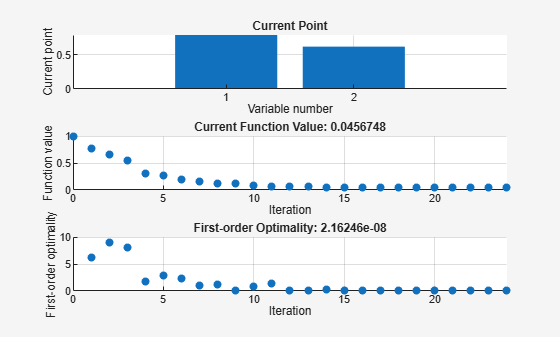## Plot Functions

### Plot an Optimization During Execution

You can plot various measures of progress during the execution of a solver. Set the `PlotFcn` name-value pair in `optimoptions`, and specify one or more plotting functions for the solver to call at each iteration. Pass a function handle or cell array of function handles.

There are a variety of predefined plot functions available. See the `PlotFcn` option description in the solver function reference page.

You can also use a custom-written plot function. Write a function file using the same structure as an output function. For more information on this structure, see Output Function and Plot Function Syntax.

### Use a Plot Function

This example shows how to use plot functions to view the progress of the `fmincon` `'interior-point'` algorithm. The problem is taken from Constrained Nonlinear Problem Using Optimize Live Editor Task or Solver.

Write the nonlinear objective and constraint functions, including their gradients. The objective function is Rosenbrock's function.

`type rosenbrockwithgrad`
```function [f,g] = rosenbrockwithgrad(x) % Calculate objective f f = 100*(x(2) - x(1)^2)^2 + (1-x(1))^2; if nargout > 1 % gradient required g = [-400*(x(2)-x(1)^2)*x(1)-2*(1-x(1)); 200*(x(2)-x(1)^2)]; end ```

Save this file as `rosenbrockwithgrad.m`.

The constraint function is that the solution satisfies `norm(x)^2 <= 1`.

`type unitdisk2`
```function [c,ceq,gc,gceq] = unitdisk2(x) c = x(1)^2 + x(2)^2 - 1; ceq = [ ]; if nargout > 2 gc = [2*x(1);2*x(2)]; gceq = []; end ```

Save this file as `unitdisk2.m`.

Create options for the solver that include calling three plot functions.

```options = optimoptions(@fmincon,'Algorithm','interior-point',... 'SpecifyObjectiveGradient',true,'SpecifyConstraintGradient',true,... 'PlotFcn',{@optimplotx,@optimplotfval,@optimplotfirstorderopt});```

Create the initial point `x0 = [0,0]`, and set the remaining inputs to empty (`[]`).

```x0 = [0,0]; A = []; b = []; Aeq = []; beq = []; lb = []; ub = [];```

Call `fmincon`, including the options.

```fun = @rosenbrockwithgrad; nonlcon = @unitdisk2; x = fmincon(fun,x0,A,b,Aeq,beq,lb,ub,nonlcon,options)``````Local minimum found that satisfies the constraints. Optimization completed because the objective function is non-decreasing in feasible directions, to within the value of the optimality tolerance, and constraints are satisfied to within the value of the constraint tolerance. ```
```x = 1×2 0.7864 0.6177 ```